# The Central Limit Theorem for Proportions Lecture 26

• Slides: 14The Central Limit Theorem for Proportions Lecture 26 Sections 8. 1 – 8. 2 Mon, Mar 3, 2008The Central Limit Theorem for Proportions n The sampling distribution of p^ is approximately normal with the following parameters.The Central Limit Theorem for Proportions n The approximation to the normal distribution is excellent if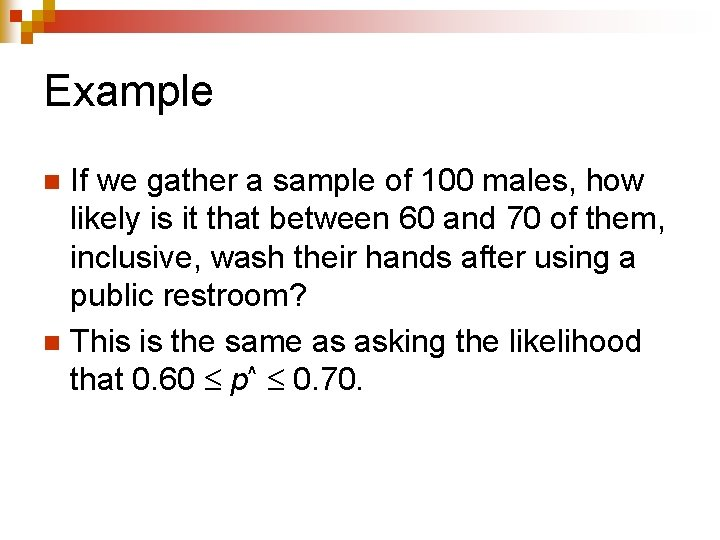Example If we gather a sample of 100 males, how likely is it that between 60 and 70 of them, inclusive, wash their hands after using a public restroom? n This is the same as asking the likelihood that 0. 60 p^ 0. 70. n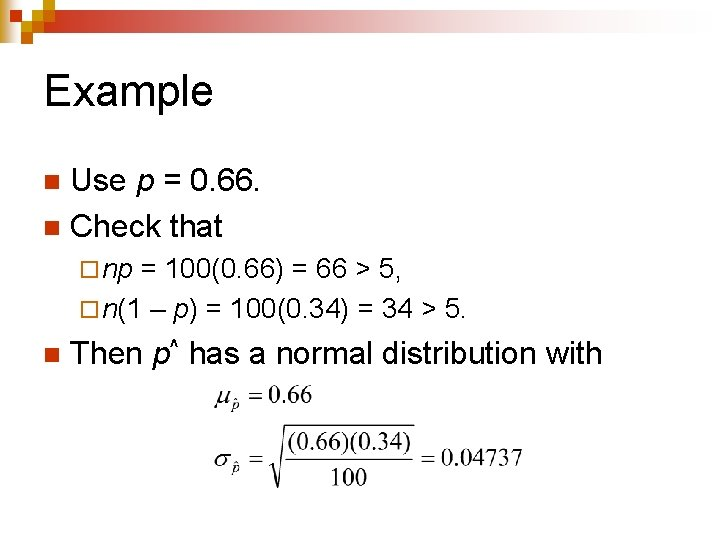Example Use p = 0. 66. n Check that n ¨ np = 100(0. 66) = 66 > 5, ¨ n(1 – p) = 100(0. 34) = 34 > 5. n Then p^ has a normal distribution with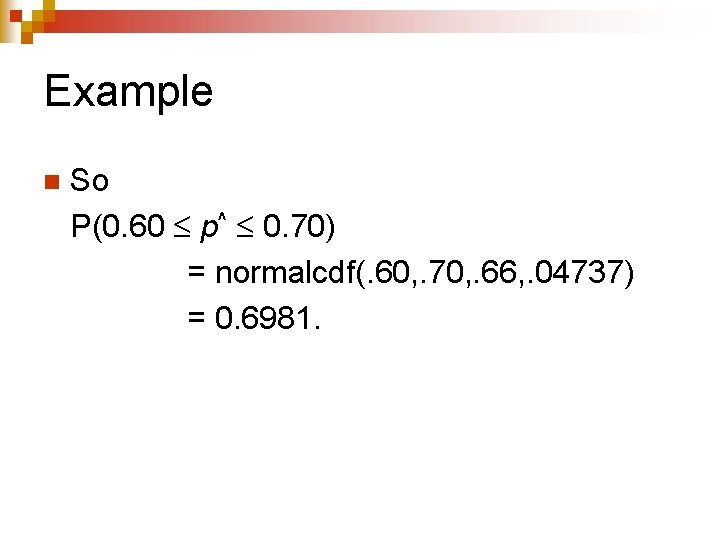Example n So P(0. 60 p^ 0. 70) = normalcdf(. 60, . 70, . 66, . 04737) = 0. 6981.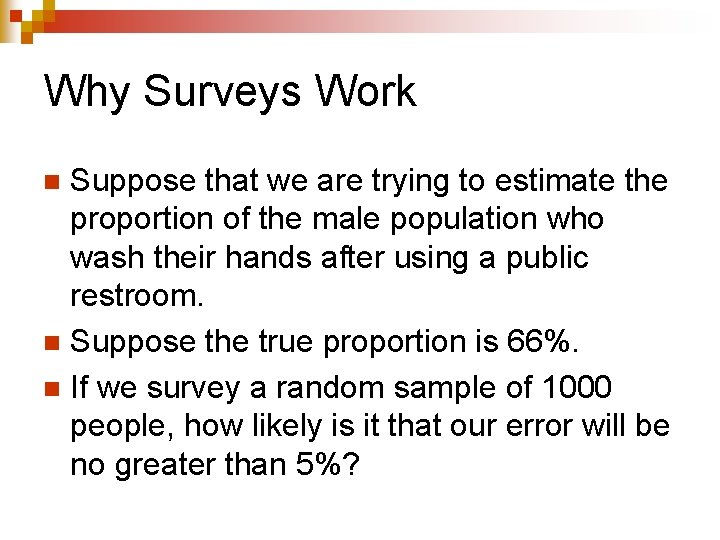Why Surveys Work Suppose that we are trying to estimate the proportion of the male population who wash their hands after using a public restroom. n Suppose the true proportion is 66%. n If we survey a random sample of 1000 people, how likely is it that our error will be no greater than 5%? nWhy Surveys Work n Now we have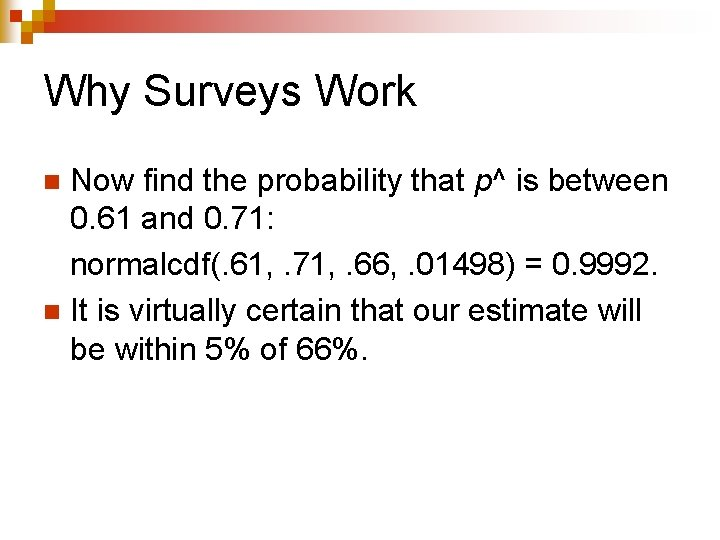Why Surveys Work Now find the probability that p^ is between 0. 61 and 0. 71: normalcdf(. 61, . 71, . 66, . 01498) = 0. 9992. n It is virtually certain that our estimate will be within 5% of 66%. n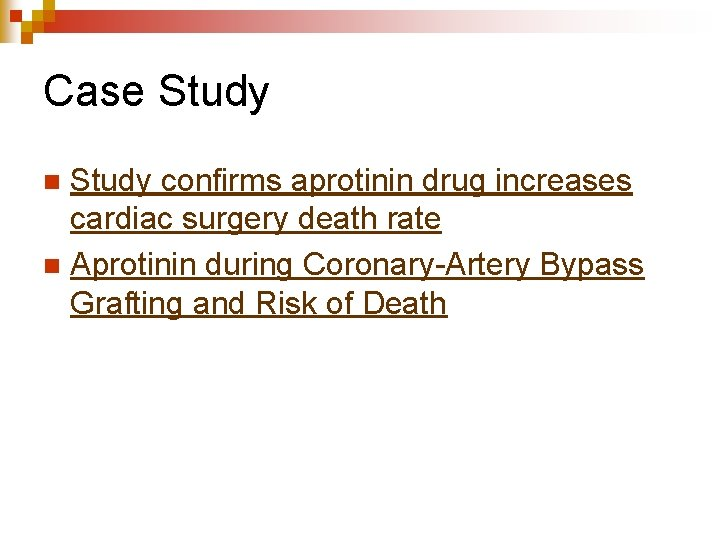Case Study confirms aprotinin drug increases cardiac surgery death rate n Aprotinin during Coronary-Artery Bypass Grafting and Risk of Death n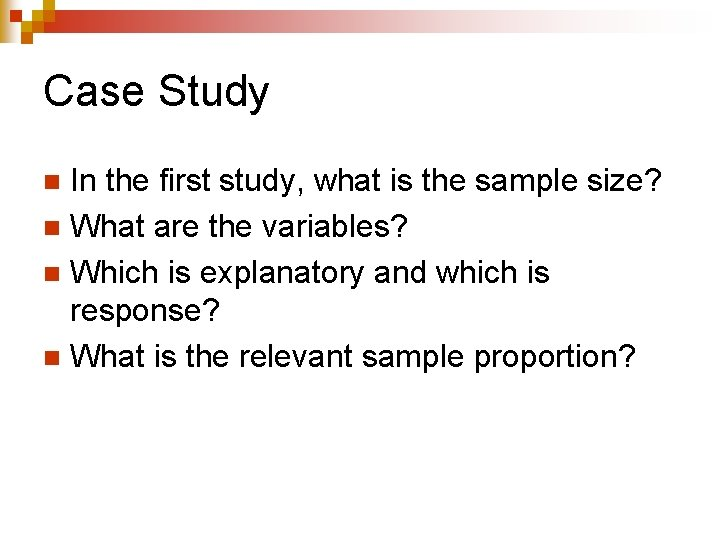Case Study In the first study, what is the sample size? n What are the variables? n Which is explanatory and which is response? n What is the relevant sample proportion? n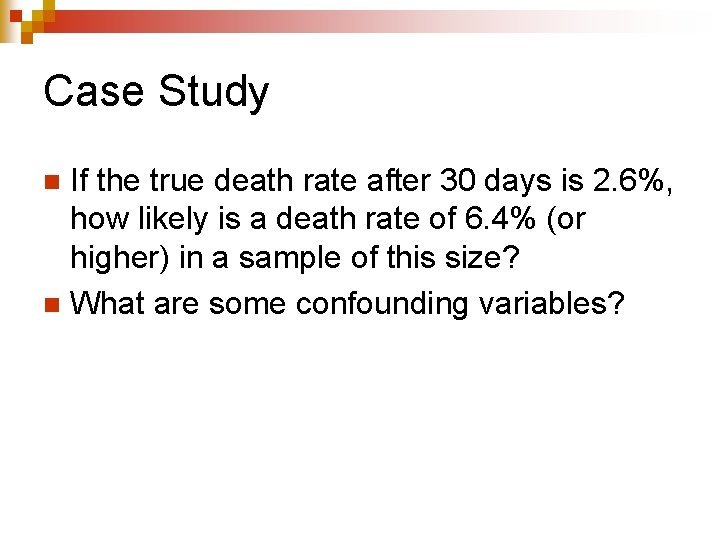Case Study If the true death rate after 30 days is 2. 6%, how likely is a death rate of 6. 4% (or higher) in a sample of this size? n What are some confounding variables? n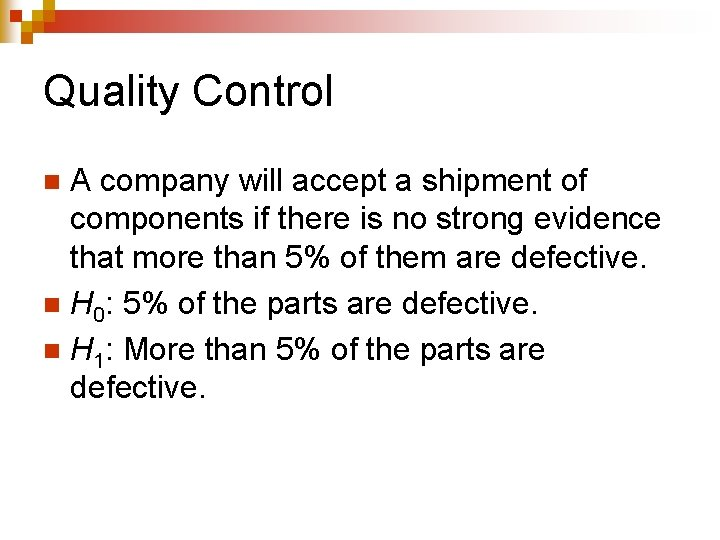Quality Control A company will accept a shipment of components if there is no strong evidence that more than 5% of them are defective. n H 0: 5% of the parts are defective. n H 1: More than 5% of the parts are defective. n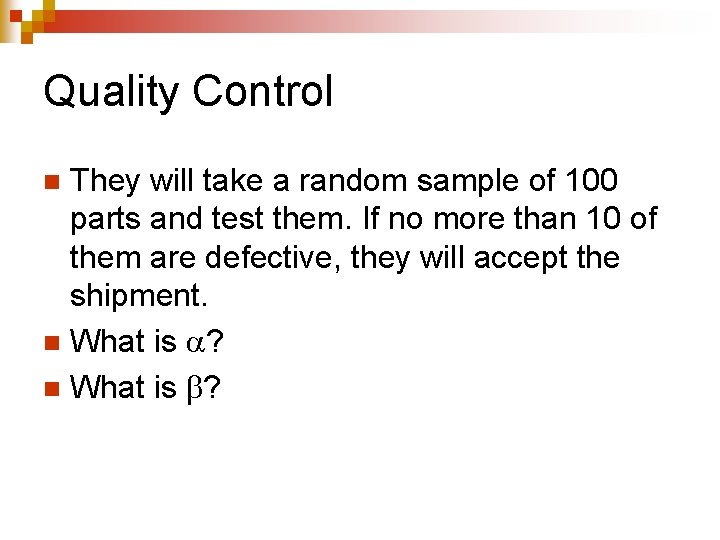Quality Control They will take a random sample of 100 parts and test them. If no more than 10 of them are defective, they will accept the shipment. n What is ? n